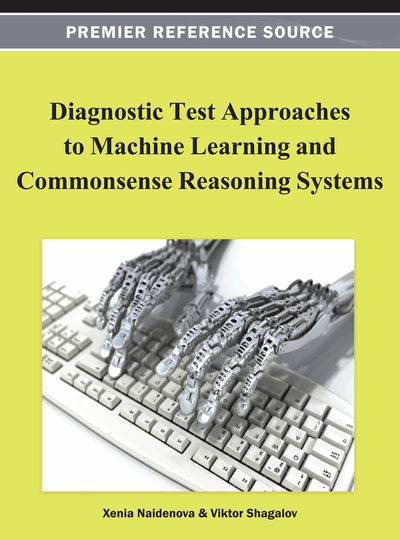# Integrated Model of Inductive-Deductive Inference Based on Finite Predicates and Implicative Regularities

DOI: 10.4018/978-1-4666-1900-5.ch001
Available
\$37.50
No Current Special Offers

## Abstract

The theory of Boolean functions, especially in respect to representing these functions in the disjunctive or conjunctive normal forms, is extended in this chapter onto the case of finite predicates. Finite predicates are decomposed by that into some binary units, which will correspond to components of Boolean vectors and matrices and are represented as combinations of these units. Further, the main concepts used for solving pattern recognition problems are defined, namely world model, data, and knowledge. The data presenting information about the existence of some objects with definite combinations of properties is considered, as well as the knowledge presenting information about the existence of regular relationships between attributes. These relationships prohibit some combinations of properties. In this way, the knowledge gives the information about the non-existence of objects with some definite (prohibited) combinations of attribute values. A special form of regularity representation, called implicative regularities, is introduced. Any implicative regularity generates an empty interval in the Boolean space of object descriptions, which do not contradict the data. The problem of plausibility evaluation of induced implicative regularities should be solved by that. The pattern recognition problem is solved by two steps. First, regularities are extracted from the database (inductive inference); second, the obtained knowledge is used for the object recognition (deductive inference).
Chapter Preview
Top

## Introduction

One of the most important problems of artificial intelligence is the problem of pattern recognition (Bongard, 1970; Hunt, 1975). To solve it, various formal methods were applied, usually based on the theory of Boolean functions (Triantaphyllou, 1994; Zakrevskij, 1988). However, they become insufficient when dealing with objects described in terms of multi-valued attributes, so other means should be involved in this case, finite predicates for example (Zakrevskij, 1993).

The finite predicates are two-valued functions, which arguments are variables with restricted number of values. Denote these variables by x1, x2, …, xn. Let them receive values accordingly from finite sets X1, X2, …, Xn, which direct product X1×X2××Xn generates a space M. The mapping M → {0,1} of the set M onto the two-element set {0,1} (this set is equivalent to {false, true}) is called a finite predicate.

When solving practical problems related to the usage of finite predicates, it is useful to represent the latter whenever possible in a more compact form. Here it is possible to use experience of the theory of Boolean functions, developed chiefly for the case when the considered functions are represented in the disjunctive normal form (DNF). The most efficient methods of minimization of Boolean functions and solution of logical equations are designed just for that form. It is reasonable to extend these methods onto finite predicates.

According to tradition, let us assume that an elementary conjunction k represents the characteristic function of some interval I of space M, and this interval is defined as a direct product of non-empty subsets αi, taken by one from every Xi:

I = α1×α2×...×αn, αiXi, αi ∅, i = 1, 2, ..., n.

That means that an elementary conjunction k is defined as a conjunction of several one-argument predicates xiαi (xi receives a value from subset αi) and is represented by the expression

k = (x1α1) ∧ (x2α2) ∧ ... ∧ (xnαn).

The multiplicands, for which αi=Xi (in this case predicate xiαi becomes identical to true), may be dropped.

Note, that in the simplest case, when all arguments become two-valued, this definition coincides with the definition of elementary conjunction in Boolean algebra.

Similarly, we shall define an elementary disjunction d as a disjunction of one-argument predicates distinct from true:

d = (x1α1) ∨ (x2α2) ∨ ... ∨ (xnαn), αiXi, i = 1,2,...,n.

If αi= ∅, the term xiαi can be deleted from any elementary disjunction, as representing the identically false expression.

The disjunctive and conjunctive normal forms are defined as usual: DNF is a disjunction of elementary conjunctions, and CNF is a conjunction of elementary disjunctions.

## Complete Chapter List

Search this Book:
Reset